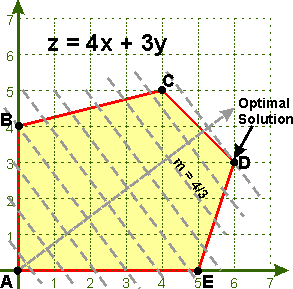# 5.6 - Linear Programming

In business, it is often desirable to find the production levels that will produce the maximum profit or the minimum cost. The production process can often be described with a set of linear inequalities called constraints. The profit or cost function to be maximized or minimized is called the objective function. The process of finding the optimal levels with the system of linear inequalities is called linear programming (as opposed to non-linear programming).

## Definitions

Objective Function
The linear function (equal sign) representing cost, profit, or some other quantity to be maximized of minimized subject to the constraints.
Constraints
A system of linear inequalities.
Problem Constraints
The linear inequalities that are derived from the application. For example, there may be only 40 hours a machine can be used in a week, so the total time it is used would have to be <= 40. The problem constraints are usually stated in the story problem.
Non-Negativity Constraints
The linear inequalities x>=0 and y>=0. These are included because x and y are usually the number of items produced and you cannot produce a negative number of items, the smallest number of items you could produce is zero. These are not (usually) stated, they are implied.
Feasible Region
The solution to the system of linear inequalities. That is, the set of all points that satisfy all the constraints. Only points in the feasible region can be used.
Corner Point
A vertex of the feasible region. Not every intersection of lines is a corner point. The corner points only occur at a vertex of the feasible region. If there is going to be an optimal solution to a linear programming problem, it will occur at one or more corner points, or on a line segment between two corner points.
Bounded Region
A feasible region that can be enclosed in a circle. A bounded region will have both a maximum and minimum values.
Unbounded Region
A feasible region that can not be enclosed in a circle.

## Fundamental Theorem of Linear Programming

Recall that almost every area of mathematics has its fundamental theorem.

Here are some of the fundamental theorems or principles that occur in your text.

Fundamental Theorem of Arithmetic (pg 8)
Every integer greater than one is either prime or can be expressed as an unique product of prime numbers.
Fundamental Theorem of Algebra (pg 264)
Every polynomial in one variable of degree n > 0 has at least one real or complex zero.
Fundamental Counting Principle (pg 543)
If there are m ways to do one thing, and n ways to do another, then there are m*n ways of doing both.

### Fundamental Theorem of Linear Programming

If there is a solution to a linear programming problem, then it will occur at a corner point, or on a line segment between two corner points.

The Fundamental Theorem of Linear Programming is a great help. Instead of testing all of the infinite number of points in the feasible region, you only have to test the corner points. Whichever corner point yields the largest value for the objective function is the maximum and whichever corner point yields the smallest value for the objective function is the minimum.

## Solving a Linear Programming Problem

If the problem is not a story problem, skip to step 3.

1. Define the variables. Usually, a good choice for the definition is the quantity they asked you to find in the problem.
2. Write the problem by defining the objective function and the system of linear inequalities. Don't forget about the non-negativity constraints where necessary.
3. Sketch the system of linear inequalities to obtain the feasible region.
4. Identify each corner point of the feasible region. You can find the corner points by forming a 2x2 system of linear equations from the two lines that intersect at that point and solving that system.
5. Evaluate the objective function at each corner point.
6. Choose the point yielding the largest value or smallest value depending on whether the problem is a maximization or minimization problem.

Be careful how you give the answer. The answer should give not only the maximum or minimum value (the value of the objective function), but it should also give the location where that extremum occurs. Example: The maximum value is 9 when x=2 and y=3. If it is a story problem, then give the answer in terms of the original definitions of x and y.

###Geometric Approach

If the slope of the objective function is negative and you take a line with that slope passing through the origin and move it to the right through the feasible region, the last corner point hit by that moving line will be the maximum value.

In the example shown, the last line with slope m=-4/3 that touches the feasible region touches at the corner point (6,3).

Since z=4(6)+3(3)=24+9=33, the maximum value is 33 when x=6 and y=3.

### Algebraic Approach

Now, to verify the solution non-geometrically. Since we know the optimal solution has to occur at one or more corner points, we make a table listing all the corner points and evaluate the objective function at those points.

Corner Point x y z = 4x + 3y
A 0 0 0
B 0 4 12
C 4 5 31
D 6 3 33
E 5 0 20

As you can see, the corner point with the maximum value is at (6,3).

We can also determine the minimum value from that table. A suitable answer, assuming the problem had asked for both the maximum and minimum is ...

The minimum value is 0 when x=0 and y=0.
The maximum value is 33 when x=6 and y=3.